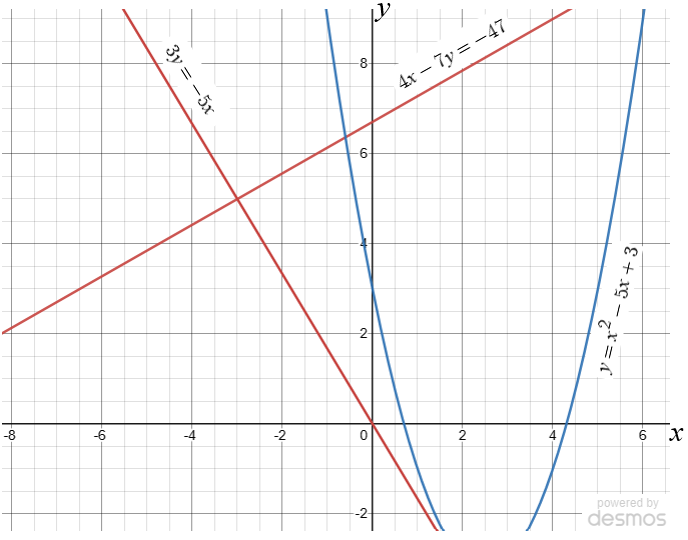# Exam-Style Questions.

## Problems adapted from questions set for previous Mathematics exams.

### 1.

GCSE Higher

Sketch the graph of $$y=0.5^x +1$$ for $$0 \le x \le 5$$ labeling the y intercept.

### 2.

GCSE Higher

(a) Use the red graphs to solve the simultaneous equations:

$$4x-7y=-47$$ $$3y=-5x$$

(b) Use the blue graph to find estimates for the solutions of the quadratic equation:

$$x^2-5x+3=0$$### 3.

GCSE Higher

The images below show a graphic display calculator screen with different functions displayed as graphs.

a) Which function is trigonometric?

b) Which function is inversely proportional to $$x$$?

c) Which function is exponential?

d) Which function is proportional to $$x^3$$?### 4.

GCSE Higher

Match the equation with the letter of its graphEquationGraph
$$y=3-\frac{10}{x}$$
$$y=2^x$$
$$y=\sin x$$
$$y=x^2+7x$$
$$y=x^2-8$$
$$y=2-x$$

### 5.

IGCSE Extended

The table shows some values (rounded to one decimal place) for the function $$y=\frac{2}{x^2}-x, x\neq 0$$.

 $$x$$ $$y$$ -3 -2 -1 -0.5 0.5 1 2 3 4 3.2 2.5 8.5 7.5 1 -2.8

(a) Complete the table of values.

(b) Draw the graph of $$y=\frac{2}{x^2}-x$$ for $$-3\le x \le -0.5$$ and $$0.5\le x\le 4$$.

(c) Use your graph to solve the equation $$\frac{2}{x^2}-x-3=0$$

(d) Use your graph to solve the equation $$\frac{2}{x^2}-x=1-2x$$

(e) By drawing a suitable tangent, find an estimate of the gradient of the curve at the point where x = 1.

(f) Using algebra, show that you can use the graph at $$y=0$$ to find $$\sqrt2$$

### 6.

GCSE Higher

(a) Find the coordinates of the point at which the curve $$y = k^x$$ intersects the y-axis.

The equation of circle $$A$$ is $$x^2+y^2=25$$. This circle is translated by the vector $$\begin{pmatrix} 0 \\ 4 \\ \end{pmatrix}$$ to give circle $$B$$.

(b) Draw a sketch of circle $$B$$ clearly labelling the points of intersection with the y-axis.

If you would like space on the right of the question to write out the solution try this Thinning Feature. It will collapse the text into the left half of your screen but large diagrams will remain unchanged.

The exam-style questions appearing on this site are based on those set in previous examinations (or sample assessment papers for future examinations) by the major examination boards. The wording, diagrams and figures used in these questions have been changed from the originals so that students can have fresh, relevant problem solving practice even if they have previously worked through the related exam paper.

The solutions to the questions on this website are only available to those who have a Transum Subscription.

Exam-Style Questions Main Page

Search for exam-style questions containing a particular word or phrase:

To search the entire Transum website use the search box in the grey area below.